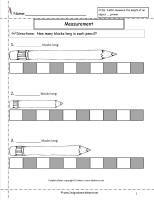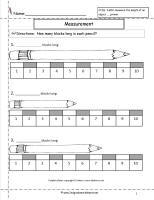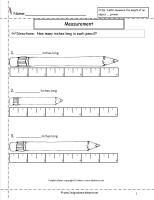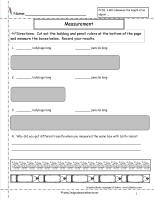﻿ CCSS 2.MD.1 Worksheets, Measuring Worksheets

# Common Core State Standard 2.MD.1

## Measurement & Data

### Measure and estimate lengths in standard units.

Measure the length of an object by selecting and using appropriate tools such as rulers, yardsticks, meter sticks, and measuring tapes.

###Measuring Worksheet

Common Core State Standards: 2.MD.1
Measurement & Data
Measure and estimate lengths in standard units.

Measure the length of an object by selecting and using appropriate tools such as rulers, yardsticks, meter sticks, and measuring tapes.

Students measure using nonstandard units.

###Measuring Worksheet

Common Core State Standards: 2.MD.1
Measurement & Data
Measure and estimate lengths in standard units.

Measure the length of an object by selecting and using appropriate tools such as rulers, yardsticks, meter sticks, and measuring tapes.

Students measure using nonstandard units.

###Measuring Worksheet

Common Core State Standards: 2.MD.1
Measurement & Data
Measure and estimate lengths in standard units.

Measure the length of an object by selecting and using appropriate tools such as rulers, yardsticks, meter sticks, and measuring tapes.

Students measure using nonstandard units.

###Measuring Worksheet

Common Core State Standards: 2.MD.1
Measurement & Data
Measure and estimate lengths in standard units.

Measure the length of an object by selecting and using appropriate tools such as rulers, yardsticks, meter sticks, and measuring tapes.

Students measure using nonstandard units.

###Measuring Worksheet

Common Core State Standards: 2.MD.1
Measurement & Data
Measure and estimate lengths in standard units.

Measure the length of an object by selecting and using appropriate tools such as rulers, yardsticks, meter sticks, and measuring tapes.

Students measure using nonstandard units.

###Measuring Worksheet

Common Core State Standards: 2.MD.1
Measurement & Data
Measure and estimate lengths in standard units.

Measure the length of an object by selecting and using appropriate tools such as rulers, yardsticks, meter sticks, and measuring tapes.

Students measure using inches.

###Measuring Worksheet

Common Core State Standards: 2.MD.1
Measurement & Data
Measure and estimate lengths in standard units.

Measure the length of an object by selecting and using appropriate tools such as rulers, yardsticks, meter sticks, and measuring tapes.

Students measure using inches.

###Measuring Worksheet

Common Core State Standards: 2.MD.1
Measurement & Data
Measure and estimate lengths in standard units.

Measure the length of an object by selecting and using appropriate tools such as rulers, yardsticks, meter sticks, and measuring tapes.

Students measure using two different units.

###Measuring Worksheet

Common Core State Standards: 2.MD.1
Measurement & Data
Measure and estimate lengths in standard units.

Measure the length of an object by selecting and using appropriate tools such as rulers, yardsticks, meter sticks, and measuring tapes.

Students choose appropriate tool to measure objects.